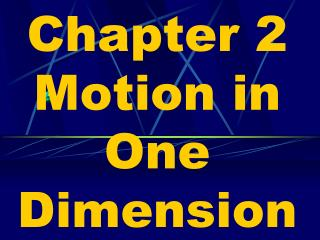# Chapter 2 Motion in One Dimension - PowerPoint PPT PresentationDownload PresentationChapter 2 Motion in One Dimension

Chapter 2 Motion in One DimensionDownload Presentation## Chapter 2 Motion in One Dimension

- - - - - - - - - - - - - - - - - - - - - - - - - - - E N D - - - - - - - - - - - - - - - - - - - - - - - - - - -
##### Presentation Transcript

1. Chapter 2 Motion in One Dimension

2. Motion is relative.

3. An object can be moving with respect to one object and at the same time be at rest or moving at a different speed with respect to another.

4. Frame of Reference • Is the point with which a motion is described.

5. How fast are you moving at this moment?

6. Depends upon how you look at it? If you look at it from the point of view with the room, most of you are not moving.

7. If you look at it from the point of view from outer space, then you are moving as fast as the earth is rotating.

8. Or from out side the solar system, the earth is moving around the sun at a speed of approximately 100,000 km/hr.

9. Relative Motion Animation

10. Vector A physical quantity that has both magnitude and direction.

11. Ex: 10 km, North 15 m/s, SW

12. Scalar A physical quantity that has magnitude, but no direction.

13. Ex: 55 km/hr 19 m

14. Distance How far an object has moved. No direction, therefore a scalar. Ex: 20 km

15. Displacement The change in position of an object

16. Difference b/n Distance & Displacement

17. Displacement = Change in position = Final position – Initial position

18. D x = xf – xi

19. Note: Displacement is not always equal to distance moved.

20. Displacement can be positive (+) or negative (-).

21. On the x-axis displacement to the right is (+) and displacement to the left is (-). On the y-axis displacement upwards is (+) and displacement downwards is (-).

22. Speed Measure of how fast something is moving.

23. Is the distance covered per unit of time. ex: 72 km/hr or 20 m/s

24. Speed Units • m/s, km/hr, or cm/s, same as velocity units. • Since speed has no direction, it is a scalar.

25. The fast speed possible is the speed of light. Which is 3 x 108 m/s (299,792,458 m/s)

26. Instantaneous Speed • Is the speed of an object at any instant.

27. Instantaneous Speed • Is the speed of an object at any instant. • Ex: speedometer reading

28. Average Speed The total distance divided by the time interval during which the displacement occurred. (Vavg)

29. Change in position Vavg = ---------------------- Change in time total distance = ----------------------- time interval

30. D Xvavg = ------D t

31. xf – xivavg = --------- Dt

32. x D v t D avg

33. The cheetah averages 70 m/s over 30 seconds. How far does it travel in those 30 seconds?

34. Velocity Is the rate of change of displacement. • It is speed with a direction. (vector)

35. Three Ways to Change Velocity

36. Three Ways to Change Velocity

37. Ex 1: During a trip, a plane flies directly East with an average velocity of 35 m/s. What distance does the plane cover in 45 minutes?

38. G: Vavg = 35 m/s, D t= 45 min= 2700 s U:D X = ? E: D X = (Vavg)(D t) S: DX=(35m/s)(2700s) S: D X = 94,500 m, E

39. With your group, work together to solve practice problems: 2, 4, and 6 on page 44 (HP). Do these problems in your notes. I will check for them in the notebook check. Also use the GUESS method.

40. 2) 3.1 km 4) 3 hr 6a) 6.4 Hour 6b) 77 km/hr South

41. Velocity is not the same as speed.

42. Uniform Motion Both velocity/speed and direction of the body/object remain the same.

43. Accelerated Motion • Is when the velocity/speed of the object is changing.

44. Acceleration (aavg) Is the rate of change of velocity. How fast you change your velocity

45. How do you know your accelerating?

46. How do you know your accelerating?

47. aavg has direction and magnitude; therefore, it is a vector.

48. Change in Velocity aavg = --------------- Time interval for change